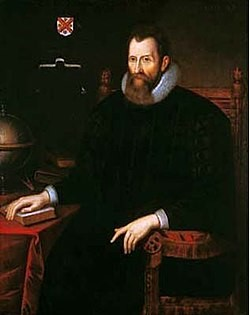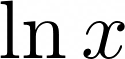## NapierJohn Napier of Merchiston (1 February 1550 – 4 April 1617), nicknamed Marvellous Merchiston, was a Scottish landowner known as a mathematician, physicist, and astronomer. He was the 8th Laird of Merchiston. His Latinized name was Ioannes Neper.

John Napier is best known as the discoverer of logarithms. He also invented the so-called “Napier’s bones” and made common the use of the decimal point in arithmetic and mathematics.

His work, Mirifici Logarithmorum Canonis Descriptio (1614) contained fifty-seven pages of explanatory matter and ninety pages of tables of numbers related to natural logarithms (see Napierian logarithm). The book also has an excellent discussion of theorems in spherical trigonometry, usually known as Napier’s Rules of Circular Parts.

The natural logarithm of a number is its logarithm to the base of the mathematical constant e, where e is a transcendental number approximately equal to 2.718281828459. The natural logarithm of x is generally written asor.